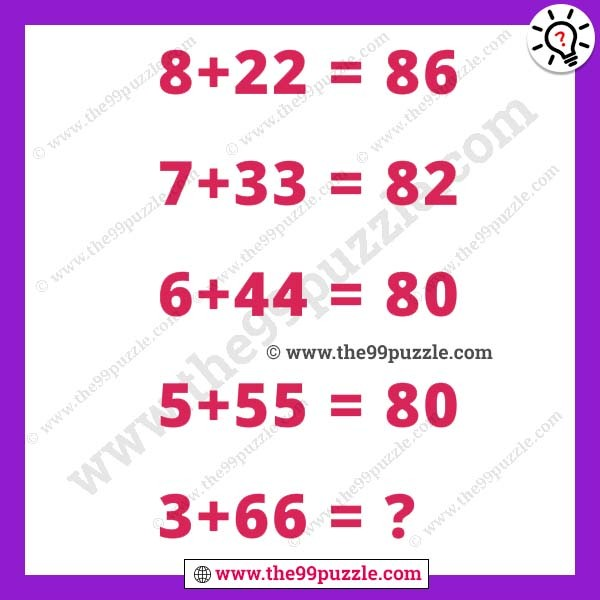# Logic maths puzzle with answer – Puzz246

Only genius solves this quick math brain teaser. The mathematical puzzle is one type of brain game. This logic maths puzzle can crack your mind. Puzzle lovers like to solve various types of brain teasers puzzles. Practice more puzzles that you make more genius and smarter. All mathematical puzzle questions have math tricks. You have to crack the math tricks and easily solve the puzzles. In this math puzzle, you see some tricky math equations. If you are a genius in mathematics you can quickly solve this puzzle.

8+22 = 86

7+33 = 82

6+44 = 80

5+55 = 80

3+66 = ?The answer will be updated very soon

Here you will find many , and many more puzzles. We are creating various fun and interesting types of puzzles for adults and kids. Logic maths puzzle with answer. Always think logically to solve easily these types of puzzles. On this website, you will play very fun games & quizzes that can improve your visual and logical thinking. Regularly we are update new puzzles & quizzes on this site. Check out daily to get new puzzles, riddles & quizzes.